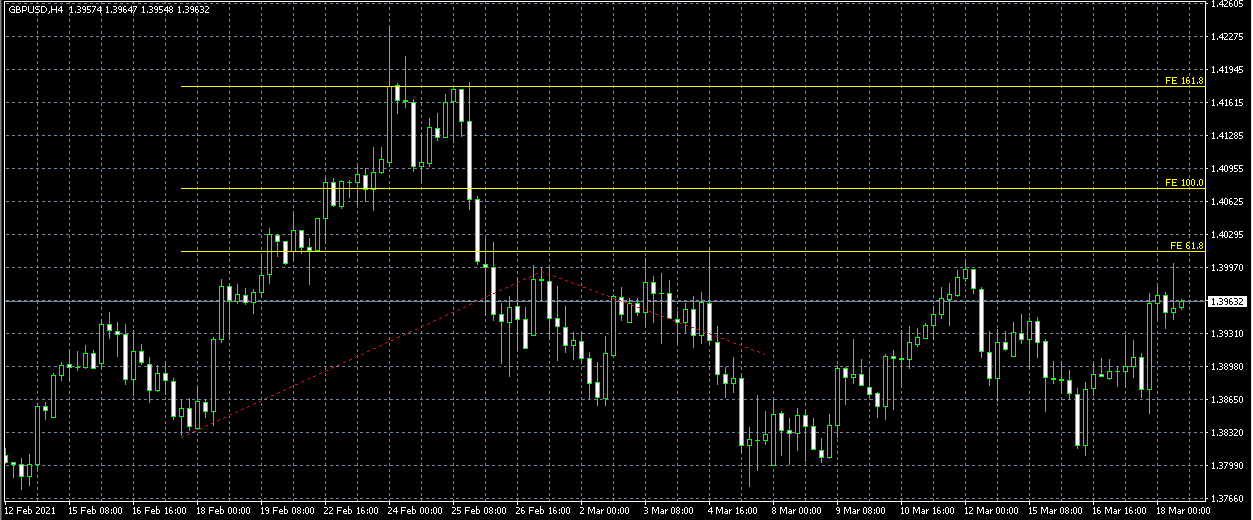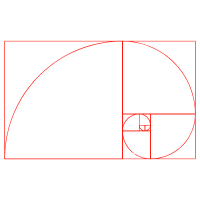# Using Fibonacci Extensions – Can it be helpful?

Fibonacci extensions are very popular indicators that are used by traders to establish certain profit targets. Many traders also use it to predict how far a price can go after a retracement. Traders also regard the extension levels as possible areas where the price of the currency pair, or other assets, can reverse. Extensions are used on the charts and mark the price levels which might be of significant importance. All of these different levels are based on the Fibonacci ratios.

Fibonacci is one of the most popular tools online. Using Fibonacci extensions is not hard and it can be used for different reasons. It is considered to be a universal trading tool that can be used for all timeframes and markets.

## Fibonacci extensions definition – How do they work?

There is no specific formula for Fibonacci extensions. When you apply the extension to the chart, you will choose three points. After this, you will see the lines drawn on the chart, which are created according to the percentages of the move.

The first point that is chosen is the start of the move, the second point is the end of the move, while the third point is at the end of the retracement against that move. After this, the extensions are capable of projecting where the price might go next.

Forex Fibonacci extension is a great way to establish price targets and find projected areas of support and resistance levels when the price is in a condition where it is very hard to find the support and resistance levels using other methods. However, it should also be noted that Fibonacci extensions are areas of possible interest.

Thus, the price may not stop or change at the exact level, however, the area around the level is of great importance. So, let’s say that you are trading an asset with a long position and you see that a new high has been reached. In this case, you can use the Fibonacci extension and see possible directions the market might take. The same is true for those who have short positions. A great thing about this indicator is that it can be used in many different situations.In the picture shown above, you can see Fibonacci Extention applied to the GBP/USD Chart. As you can see, there are three levels of Fibonacci Extension shown in the candlestick chart – 161.8; 100,0; and 61.8.

### Forex Fibonacci extension levels

Fibonacci extensions are very frequently used by traders around the world. They are most frequently used to determine take profit levels during a certain trend. In the market, you will come across different types of Fibonacci extension levels, however, the most commonly used ones are 138.2 and 161.8.

There are other common Fibonacci extension levels as well, such as 61.8%, 100%, 200%, and 261.8%. For many, Fibonacci extension levels Forex trading is a very important tool, as they believe that it can help them understand how far a certain trend can go after a pullback.

Traders can easily get access to the Fibonacci extension through different types of trading platforms. There is a Fibonacci indicator for MT4, MT5, cTrader, and many other trading platforms. This makes it easy for everyone to understand and for many traders, it is a key component of technical analysis.

## How can Fibonacci extensions be calculated?

Before we learn how Fibonacci extensions Forex is calculated, let’s first understand how to calculate the basic Fibonacci sequence. To put it simply, it begins with 0 and 1, after which, it creates the third number by adding the two previous numbers. So, this is how it looks: 0, 1, 1, 2, 3, 5, 8, 13, 21, 34, 55, 89, and so on.As you might notice, every number within the sequence gets closer and closer to 61.8% of the following number. On the other hand, every number in the sequence gets closer to the 38.2% of the number two positions after it, and 23.6% of the number three positions after it.

To understand the Fibonacci Forex ratio, learning the details about Fibonacci can be a great help. Another number that has an impact on the price action is 100%, although it is not a Fibonacci number. As for the Fibonacci number relationships above 100, they are calculated using the same methods, however, in reverse order.

Because of this, as we have already said earlier in the guide, the primary Fibonacci numbers above 100 include 123.6%, 138.2%, 150%, 161.8%, and 261.8%. You can remember these numbers by simply adding 100 to the standard Fibonacci levels. In most cases, traders use Fibonacci retracements for moves that are within the trend.

After this, they tend to switch to the Fibonacci extensions when the price goes through the 100% Fibonacci level of the base trend. In most cases, this is a sign that the reversal might be of a larger magnitude than the base trend was itself.

## Fibonacci extension MT4 indicator

Most of the trading platforms, including MetaTrader 4, offer traders pre-installed Fibonacci extension indicators. It is very simple to use, and you can add it to your chart in just a few clicks. The first thing that you will have to do to add the Fibonacci extension to your chart is to go to the Insert, then go to Fibonacci, and choose Extension. After this, you will be able to choose the points that you want to and see the results in no time.The picture above shows how you can add the Fibonacci Extention to your charts using MetaTrader 4. The same methods can be used for MetaTrader 5.

The first thing that you need to do to draw a Fibonacci extension is to find a trend you want to use as a base. After clicking the beginning and the end of the trend, you should be able to see the result.  Fibonacci extension MT5 works exactly the same way and can be used for various asset classes, on different time frames.

## Final thoughts on trading Fibonacci Extensions

Fibonacci extensions represent a very important part of technical analysis. They are used by millions of traders and can be very helpful to identify possible support and resistance levels. Fibonacci Forex indicators can be easily accessed with the help of trading platforms. It is available on MT4, MT5, cTrader, and many others.

The best thing about Fibonacci extensions is that they can be used very easily by anyone. In just a few clicks it can be added to the chart, which makes it very helpful for traders of all backgrounds and experience.

How do you use Fibonacci extensions?

Fibonacci extensions can be determined in just a few clicks. When you open the trading platform, you will have to choose the Fibonacci extension from the list of indicators. After this, you will have to determine the levels using three mouse clicks. First, you click on the beginning of the trend, after which, you choose the end of the trend. Finally, you drag the cursor back down and click on any of the retracement levels. After this, you can see the final result.

Does MT4 have Fibonacci?

In most cases, MetaTrader 4 comes with built-in Fibonacci indicators. If you are can’t find it among the indicators on your MT4, you can find it online, download it, and add it to your trading platform. Using Fibonacci extensions on MT4 is very easy and simple. All you have to do is to open your chart, then, on the top of the menu choose Insert, then go to Fibonacci, and choose extensions that you are good to go.